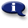# .

## Syllabus Information

Fall 2022
May 31,2023Use this page to maintain syllabus information, learning objectives, required materials, and technical requirements for the course.

Syllabus Information
MTH 070 - Introductory Algebra
Associated Term: Fall 2022
Learning Objectives: Upon successful completion of this course, the student will be able to:
1. Maintain, use, and expand skills and concepts learned in previous mathematics courses
a. Perform operations with fractions and decimals
b. Use unit analysis to convert units and solve problems
2. Perform addition, subtraction, multiplication, and division of rational numbers
3. Use and apply the concepts and language of algebraic expressions
a. Use variables to construct algebraic expressions
b. Evaluate algebraic expressions and simplify expressions using order of operations
c. Simplify algebraic expressions by removing parentheses and combining like terms
4. Solve linear equations and inequalities
a. Solve linear equations and formulas algebraically
b. Solve linear inequalities and graph their solutions on a number line and using interval notation
5. Use algebra to solve application problems
a. Translate verbal models into algebraic expressions and/or equations. Solve problems
b. Solve problems using ratios and proportions
c. Solve problems involving similar triangles
d. Solve geometry problems involving perimeter, area, and volume
6. Interpret information, represented numerically and graphically, and recognize linear relationships represented verbally, numerically, graphically, and algebraically
a. Read and interpret information given in a table or from a graph
b. Locate points in a rectangular coordinate system and represent equations in two variables graphically
c. Identify the horizontal and vertical intercepts of the graph of an equation and interpret them in terms of an application
d. Identify the slope of a line and interpret it in terms of an application
e. Use the slope-intercept form of the equation of a line
f. Use the point-slope form of the equation of a line
g. Recognize the relationship between the slopes of two lines that are parallel or perpendicular
7. Solve linear systems of two equations algebraically and graphically
a. Solve algebraically and by graphing
b. Solve application problems involving linear systems of equations (including simple interest, motion, and mixture problems)
8. Evaluate and/or simplify expressions using the rules of exponents
9. Use scientific notation
10. Use the terminology of polynomials and add, subtract, multiply and divide polynomials
a. Recognize and use the terminology of polynomials
b. Evaluate polynomials
c. Add, subtract, multiply, and divide (by a monomial) polynomials
11. Factor polynomials
a. Factor polynomials by removing a common monomial factor
b. Factor by grouping
c. Factor trinomials
d. Factor special products
12. Recognize and use quadratic equations
a. Sketch the graph of a quadratic equation in two variables and identify the intercepts and vertex graphically
b. Solve a quadratic equation by factoring
c. Solve applications by writing and solving quadratic equations (by factoring), including problems involving the Pythagorean Theorem
13. Understand the basic definition of a function
a. Recognize and interpret function notation
b. Evaluate functions using function notation
c. Find the domain of a function defined by a table or list of ordered pairs
14. Recognize and use rational expressions
a. Recognize values of a variable that make a rational expression undefined
b. Reduce rational expressions to lowest terms
c. Multiply and divide rational expressions
d. Add and Subtract rational expressions with like denominators
15. Make appropriate and efficient use of a scientific calculator
Required Materials:
Technical Requirements: